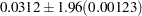Output Data Sets

### OUT= Data Set

The OUT= data set contains contrast names (_test_), variable names (_var_), the contrast label (_contrast_), raw p-values (raw_p or the value specified in the INPVALUES= option), and all requested adjusted p-values (bon_p, sid_p, boot_p, perm_p, stpbon_p, stpsid_p, stpbootp, stppermp, hom_p, hoc_p, fic_p, stouffer_p, aholm_p, ahoc_p, fdr_p, dfdr_p, fdrbootp, ufdbootp, fdrpermp, ufdpermp, afdr_p, or pfdr_p).

If a resampling-based adjusted p-value is requested, then the simulation standard error is included as either sim_se, stpsimse, fdrsimse, or ufdsimse, depending on whether single-step, step-down, or FDR adjustments are requested. The simulation standard errors are used to bound the true resampling-based adjusted p-value. For example, if the resampling-based estimate is 0.0312 and the simulation standard error is 0.00123, then a 95% confidence interval for the true adjusted p-value is, or 0.0288 to 0.0336.

Intermediate statistics used to calculate the p-values are also written to the OUT= data set. The statistics are separated by the _strat_ level. When _strat_ is reported as missing, the statistics refer to the pooled analysis over all _strat_ levels. The p-values are provided only for the pooled analyses and are therefore reported as missing for the strata-specific statistics.

For the Peto test, an additional variable, _tstrat_, is included to indicate whether the stratum is an incidental occurrence stratum (_tstrat_=0) or a fatal occurrence stratum (_tstrat_=1).

The statistic _value_ is the per-strata contribution to the numerator of the overall test statistic. In the case of the MEAN test, this is the contrast function of the sample means multiplied by the total number of observations within the stratum. For the FT test, _value_ is the contrast function of the double-arcsine transformed proportions, again multiplied by the total number of observations within the stratum. For the CA and Peto tests, _value_ is the observed value of the trend statistic within that stratum.

When either PETO or CA is requested, the variable _exp_ is included; this variable contains the expected value of the trend statistic for the given stratum.

The statistic _se_ is the square root of the variance of the per-strata _value_ statistic for any of the tests.

For MEAN tests, the variable _nval_ is included. When reported with an individual stratum level (that is, when the _strat_ value is nonmissing), the value _nval_ refers to the within-stratum sample size. For the combined analysis (that is, the value of the _strat_ is missing), the value _nval_ contains degrees of freedom of the t distribution used to compute the unadjusted p-value.

When the FISHER test is requested, the OUT= data set contains the variables _xval_, _mval_, _yval_, and _nval_, which define observations and sample sizes in the two groups defined by the CONTRAST statement.

For example, the OUT= data set from the drug example in the section Getting Started: MULTTEST Procedure is displayed in Figure 60.5.

Figure 60.5 Output Data for the MULTTEST Procedure
Obs _test_ _var_ _contrast_ _value_ _exp_ _se_ raw_p boot_p sim_se
1 CA SideEff1 Trend 8 5 1.54303 0.05187 0.33880 .003346749
2 CA SideEff2 Trend 7 5 1.54303 0.19492 0.84030 .002590327
3 CA SideEff3 Trend 10 7 1.63299 0.06619 0.51895 .003532994
4 CA SideEff4 Trend 10 6 1.60357 0.01262 0.08840 .002007305
5 CA SideEff5 Trend 7 4 1.44749 0.03821 0.24080 .003023370
6 CA SideEff6 Trend 9 6 1.60357 0.06137 0.43825 .003508468
7 CA SideEff7 Trend 9 5 1.54303 0.00953 0.05135 .001560660
8 CA SideEff8 Trend 8 5 1.54303 0.05187 0.33880 .003346749
9 CA SideEff9 Trend 7 5 1.54303 0.19492 0.84030 .002590327
10 CA SideEff10 Trend 8 6 1.60357 0.21232 0.90300 .002092737

### OUTPERM= Data Set

The OUTPERM= data set contains contrast names (_contrast_), variable names (_var_), and the associated permutation distributions (_value_ and upper_p). PROC MULTTEST computes the permutation distributions when you use the PERMUTATION= option with the CA or Peto test. The _value_ variable represents the support of the distributions, and upper_p represents their cumulative upper-tail probabilities. The size of this data set depends on the number of variables and the support of their permutation distributions.

For information about how this distribution is computed, see the section Exact Permutation Test. For an illustration, see Example 60.1.

### OUTSAMP= Data Set

The OUTSAMP= data set contains the data sets used in the resampling analysis, if such an analysis is requested. The variable _sample_ indicates the number of the resampled data set. This variable ranges from 1 to the value of the NSAMPLE= option. For each value of the _sample_ variable, an entire resampled data set is included, with _stratum_, _class_, and all other variables in the original data set. The values of the original variables are mean-centered for the mean test, if requested. The variable _obs_ indicates the observation’s position in the original data set.

Each new data set is randomly drawn from the original data set, either with (bootstrap) or without (permutation) replacement. The size of this data set is, thus, the number of observations in the original data set times the number of samples.